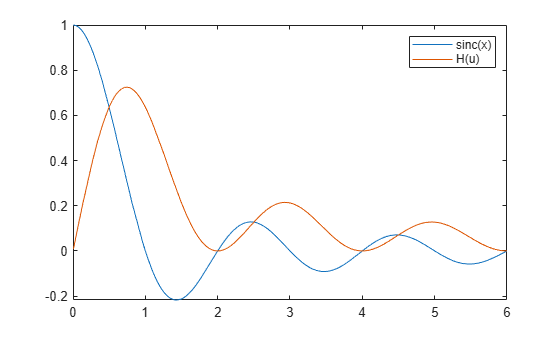# htrans

Hilbert transform

Since R2019a

## Syntax

``H = htrans(f)``
``H = htrans(f,transVar)``
``H = htrans(f,var,transVar)``

## Description

example

````H = htrans(f)` returns the Hilbert transform of symbolic function `f`. By default, the independent variable is `t` and the transformation variable is `x`.```

example

````H = htrans(f,transVar)` uses the transformation variable `transVar` instead of `x`.```

example

````H = htrans(f,var,transVar)` uses the independent variable `var` and the transformation variable `transVar` instead of `t` and `x`, respectively. If all input arguments are arrays of the same size, then `htrans` acts element-wise.If one input is a scalar and the others are arrays of the same size, then `htrans` expands the scalar into an array of the same size.If `f` is an array of symbolic expressions with different independent variables, then `var` must be a symbolic array with elements corresponding to the independent variables. ```

## Examples

collapse all

Compute the Hilbert transform of `sin(t)`. By default, the transform returns a function of `x`.

```syms t; f = sin(t); H = htrans(f)```
`H = $-\mathrm{cos}\left(x\right)$`

Compute the Hilbert transform of the `sinc(x)` function, which is equal to `sin(pi*x)/(pi*x)`. Express the result as a function of `u`.

```syms f(x) H(u); f(x) = sinc(x); H(u) = htrans(f,u)```
```H(u) =  $-\frac{\frac{\mathrm{cos}\left(\pi u\right)}{u}-\frac{1}{u}}{\pi }$```

Plot the `sinc` function and its Hilbert transform.

```fplot(f(x),[0 6]) hold on fplot(H(u),[0 6]) legend('sinc(x)','H(u)')```Create a sine wave with a positive frequency in real space.

```syms A x t u; assume([x t],'real') y = A*sin(2*pi*10*t + 5*x)```
`y = $A \mathrm{sin}\left(5 x+20 \pi t\right)$`

Apply a –90-degree phase shift to the positive frequency component using the Hilbert transform. Specify the independent variable as `t` and the transformation variable as `u`.

`H = htrans(y,t,u)`
`H = $-A \mathrm{cos}\left(5 x+20 \pi u\right)$`

Now create a complex signal with negative frequency. Apply a 90-degree phase shift to the negative frequency component using the Hilbert transform.

`z = A*exp(-1i*10*t)`
`z = $A {\mathrm{e}}^{-10 t \mathrm{i}}$`
`H = htrans(z)`
`H = $A {\mathrm{e}}^{-10 x \mathrm{i}} \mathrm{i}$`

Create a real-valued signal $\mathit{f}\left(\mathit{t}\right)$ with two frequency components, 60 Hz and 90 Hz.

```syms t f(t) F(s) f(t) = sin(2*pi*60*t) + sin(2*pi*90*t)```
`f(t) = $\mathrm{sin}\left(120 \pi t\right)+\mathrm{sin}\left(180 \pi t\right)$`

Calculate the corresponding analytic signal $\mathit{F}\left(\mathit{s}\right)$ using the Hilbert transform.

`F(s) = f(s) + 1i*htrans(f(t),s)`
`F(s) = $\mathrm{sin}\left(120 \pi s\right)+\mathrm{sin}\left(180 \pi s\right)-\mathrm{cos}\left(120 \pi s\right) \mathrm{i}-\mathrm{cos}\left(180 \pi s\right) \mathrm{i}$`

Calculate the instantaneous frequency of $\mathit{F}\left(\mathit{s}\right)$ using

`${f}_{instant}\left(s\right)=\frac{1}{2\pi }\frac{d\varphi \left(s\right)}{ds},$`

where $\varphi \left(s\right)=\mathrm{arg}\left[F\left(s\right)\right]$ is the instantaneous phase of the analytic signal.

```InstantFreq(s) = diff(angle(F(s)),s)/(2*pi); assume(s,'real') simplify(InstantFreq(s))```
`ans = $75$`

## Input Arguments

collapse all

Input, specified as a symbolic expression, symbolic function, symbolic vector, or symbolic matrix.

Independent variable, specified as a symbolic variable, symbolic vector, or symbolic matrix. This variable is usually in the time domain. If you do not specify the variable, then `htrans` uses `t` by default. If `f` does not contain `t`, then `htrans` uses the function `symvar` to determine the independent variable.

Transformation variable, specified as a symbolic variable, symbolic vector, or symbolic matrix. This variable is in the same domain as `var`. If you do not specify the variable, then `htrans` uses `x` by default. If `x` is the independent variable of `f`, then `htrans` uses the transformation variable `v`.

## Output Arguments

collapse all

Hilbert transform or harmonic conjugate of the input function `f`. The output `H` is a function of the variable specified by `transVar`.

When `htrans` cannot transform the input function, it returns an unevaluated call. To return the original expression, apply the inverse Hilbert transform to the output by using `ihtrans`.

collapse all

### Hilbert Transform

The Hilbert transform H = H(x) of the expression f = f(t) with respect to the variable t at point x is

`$H\left(x\right)=\frac{1}{\pi }\text{p}\text{.v}\text{.}\underset{-\infty }{\overset{\infty }{\int }}\frac{f\left(t\right)}{x-t}dt.$`

Here, p.v. represents the Cauchy principal value of the integral. The function f(t) can be complex, but t and x must be real.

## Tips

• To compute the inverse Hilbert transform, use `ihtrans`. The Hilbert transform of a function is equal to the negative of its inverse Hilbert transform.

• For a signal in the time domain, the Hilbert transform applies a –90-degree phase shift to positive frequencies of the corresponding Fourier components. It also applies a 90-degree phase shift to negative frequencies.

• For a real-valued signal `a`, the Hilbert transform ```b = htrans(a)``` returns its harmonic conjugate `b`. The real signal `a = real(z)` and its Hilbert transform `b = imag(z)` form the analytic signal `z = a + 1i*b`.

## Version History

Introduced in R2019a